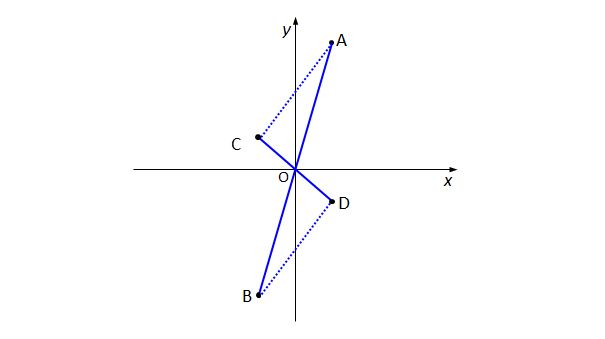Algebra

# Linear Equations - Problem Solving

The graph of $(k-1)x+y=9k+10$ always passes through the point $P=(a,b)$ regardless of the value of $k$. What is the value of $a+b$?

What is the square of the length of the line segment that lies in the first quadrant of the Cartesian plane and is part of the line that perpendicularly intersects with the line $4x-6y-21=0$ at $\left(3,-\frac{3}{2}\right)?$

For the linear function $f(x)=ax+b$, if $f(-3)=-1 \text{ and } f(x+1)-f(x-1)=20,$ what is the value of $f(15)$?Line segment $\overline{AB}$ passes through the origin $O=(0, 0)$ and the line segments $\overline{OA}$ and $\overline{OB}$ have equal lengths. Line segment $\overline{CD}$ also passes through the origin and the line segments $\overline{OC}$ and $\overline{OD}$ have equal lengths. If $A=(5, 22)$ and $C=( -5,2),$ what is the slope of the line passing through the two points $B$ and $D?$

What is the sum of all the values of $k$ that make three points $A=(1,k),$ $B=(k,1)$ and $C=(10,10)$ lie on the same line?

×